### Introduction

R has powerful graphical capability even in the R base graphics. Here I’ll give a brief tutorial for including mathematical expression in the figures. More details can be found in the help for plotmath, but it is difficult to read and comprehend, especially for beginners. I encourage you to take a look at the example and demo for plotmath first, then read the following.

demo(plotmath)
example(plotmath)

I assume - you have good understanding for most basic R plotting functions, such as plot, text, etc. - you know the Latex ways to math expressions and symbols.

The focus on this document is to add math equations to the plot.

### Quick start

The math formula in R plot needs to be wrapped in an object of expression. You can take a look at the help pages for expression, but when it is used in plot, it means to be rendered as a math formula instead of being evaluated.

Here, I’ll first create an empty figure, and then use the text function to
write a simple expression in the figure.

plot(1:10, 1:10, xlab="", ylab="", type="n")
text(5, 5, expression(f(x)==sqrt(x)))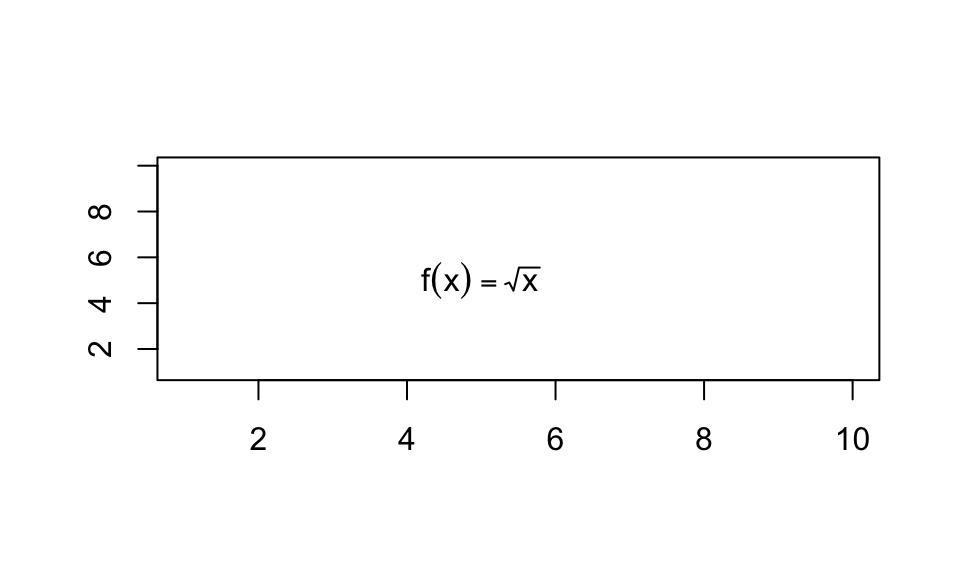A few commments on this simple example:

• The text function usually takes a string for the text. Here I give it an expression, which will be shown as a math formula in the figure.

• It’s important to note that the the content inside expression isn’t within quote marks. If you put a quote marks in the expression, it’ll become a string. You can try the following to see what you get:

plot(1:10, 1:10, xlab="", ylab="", type="n")
text(5, 5, expression("f(x)==sqrt(x)"))
• You perhaps noticed that I use things like sqrt and == in the expression, but they show as math symbols. These are the syntax R uses to render math expressions. You can get all these syntax in the help for plotmath.

### Use expressions in axis labels and figure titles

Similar to the text example above, you can use the expression in axis labels (xlab and ylab parameters) and figure titles (main parameter): Again, these parameters in the plot function usually takes strings. When you give them expressions, they will be shown as math formulas.

x = 1:100
y = sqrt(x)
plot(x, y, xlab="x", ylab=expression(sqrt(x)), main=expression(f(x)==sqrt(x)) )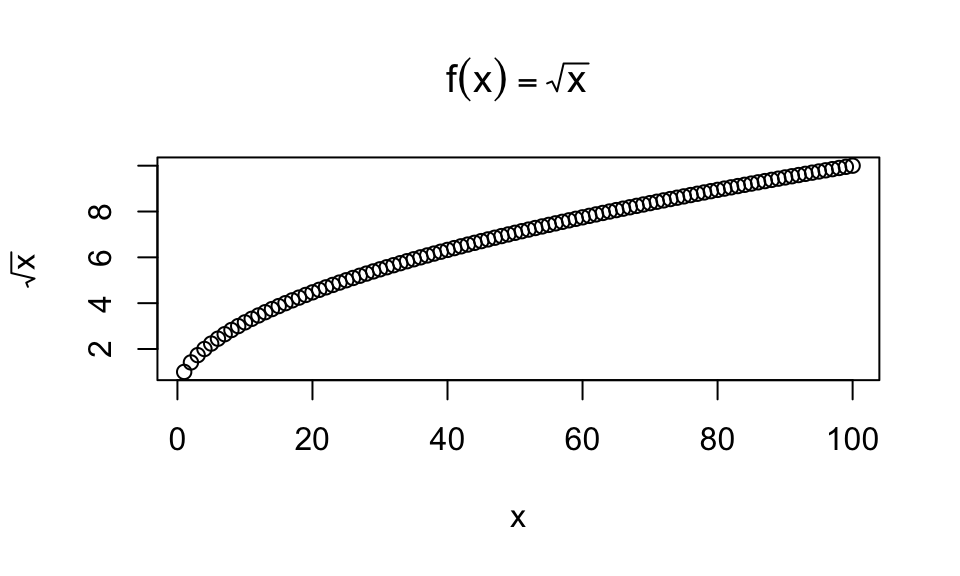### Make it a little more complicated

Of course, the math formula can be complex, and include Greek letters. This requires you to be familiar with the Latex syntax. For all possible symbols, look at demo(plotmath). The example below, I write the standard normal density function in a figure:

plot(1:10, 1:10, xlab="", ylab="", type="n")
text(5, 5, expression(f[X](x)==frac(1, sigma*sqrt(2*pi))*plain(e)^{frac(-(x-mu)^2, 2*sigma^2)}))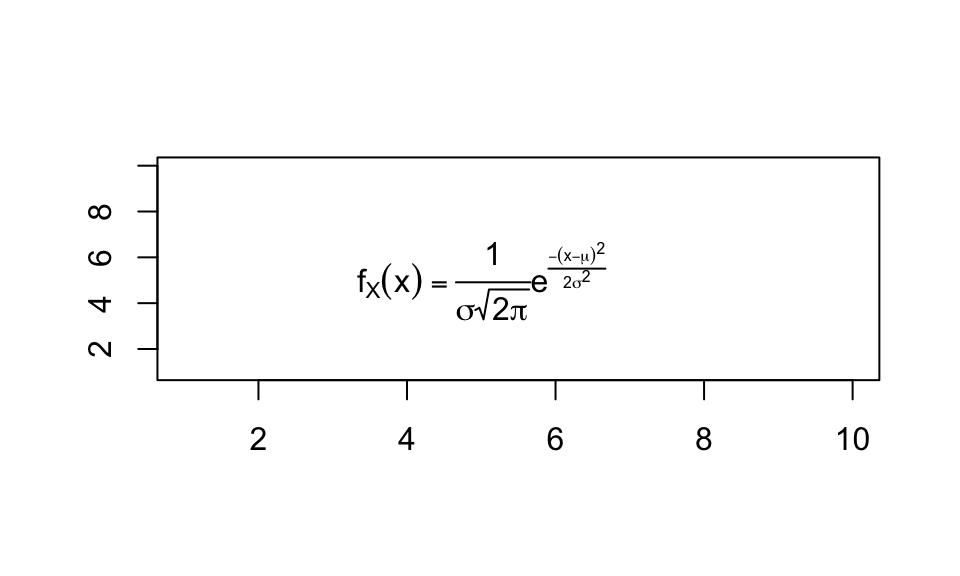• Take a careful look at the expression I used and make sure you understand all the parts.
• Note, the expression, albeit based on Latex, has some differences. For example, the subscript in Latex is achieved by underscore, i.e., f_X is shown as $$f_X$$ in latex. But in R, since the underscore is a reserved symbol, you must use f[X] in the expression to display $$f_X$$.

### Use expression with paste

Sometimes, you want to mix math expressions with simple text. For that, you have to use paste function to put the expression and text together.

Here is a simple example:

plot(1:10, 1:10, xlab="", ylab="", type="n")
text(5, 5, expression(paste("My function is ", f(x)==sqrt(x))) )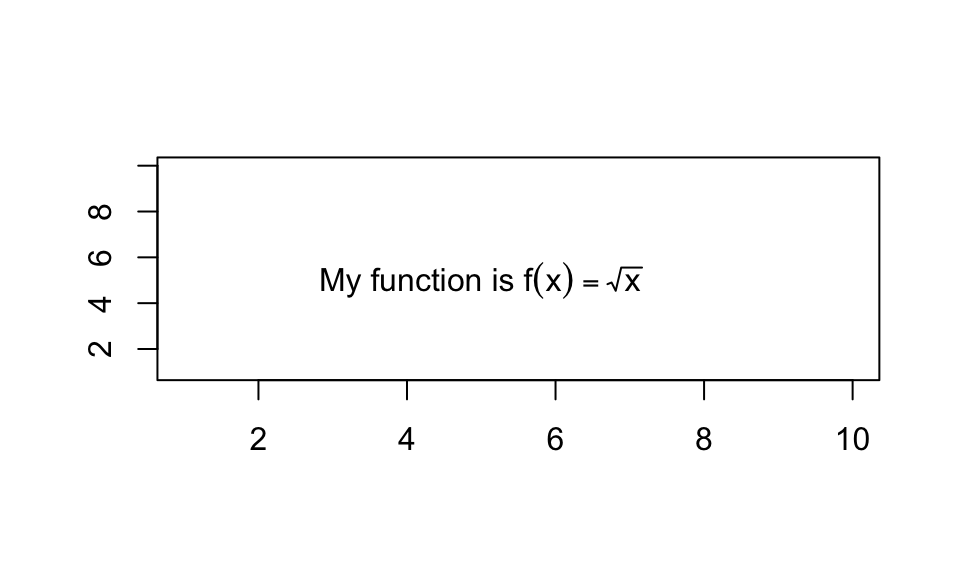A few notes:

• You must use expression outside of paste, so that the things pasted together will be evaluated as an expression. If you reverse the order, the figure will be messed up. Try following:

plot(1:10, 1:10, xlab="", ylab="", type="n")
text(5, 5, paste(expression("My function is ", f(x)==sqrt(x))) )
• paste is usually used for concatenating strings together, but here it takes a string and an expression.

• If you want to have some R reserved special characters in the expression, for exampe, semi-colon, underscore, even space, you have to paste them in. For example, if you want to display a formula $$f(x;k) = x^k$$, you cannot do the following (you can try, it’ll give you an error).

plot(1:10, 1:10, xlab="", ylab="", type="n")
text(5, 5, expression(f(x;k) = x^k) )

You’ll need to paste the semi-colon in:

plot(1:10, 1:10, xlab="", ylab="", type="n")
text(5, 5, expression(paste("f(x;k) = ", x^k)))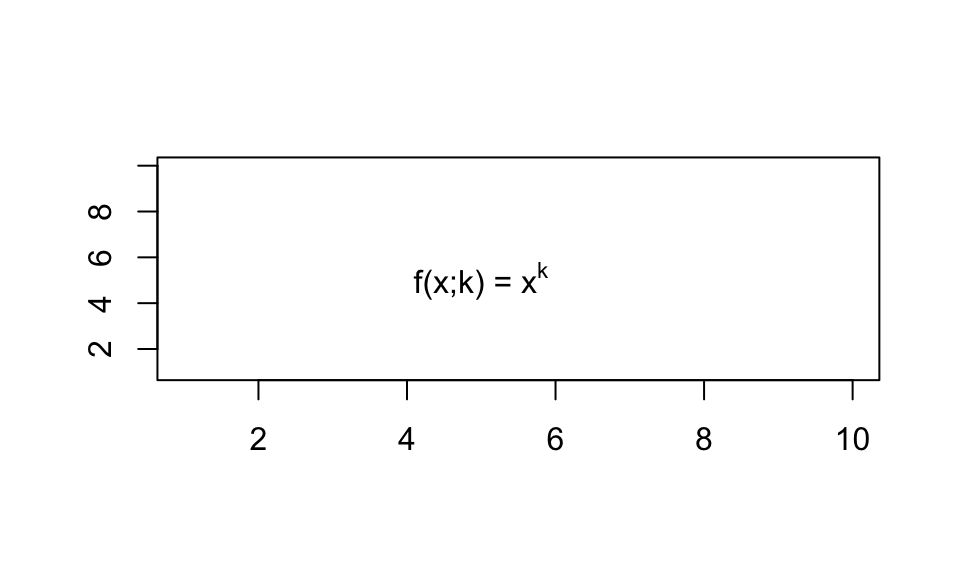A more complicated example. If you want to display $$f(x; \sigma) = x^\sigma$$, Since the greek letter $$\sigma$$ need to be typed in as sigma in the expression, you need to paste several components together.

plot(1:10, 1:10, xlab="", ylab="", type="n")
text(5, 5, expression(paste("f(x;", sigma, ") = ", x^sigma)))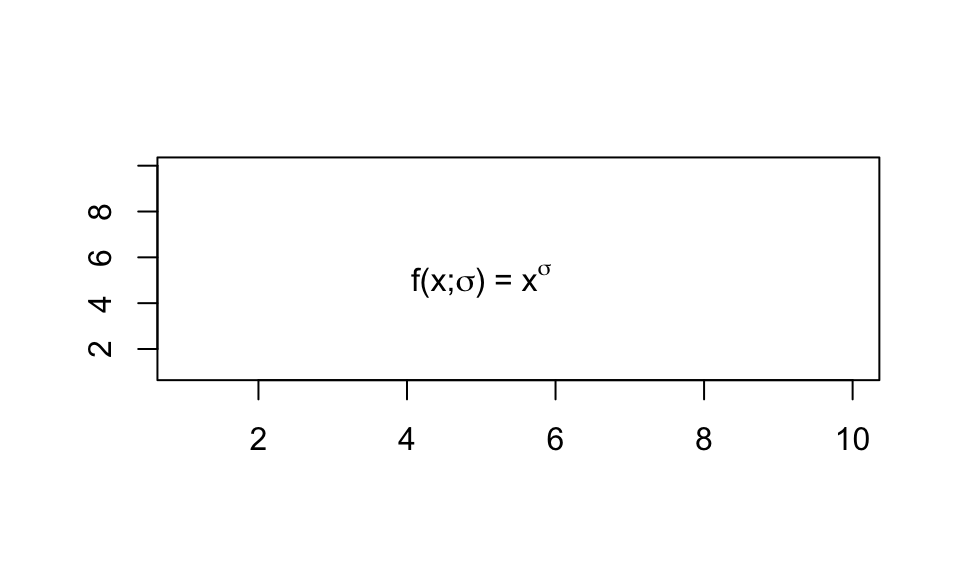In the above expression, I pasted 4 parts together:

• "f(x;" as a string
• sigma as an expression
• ") = " as a string
• x^sigma as an expression